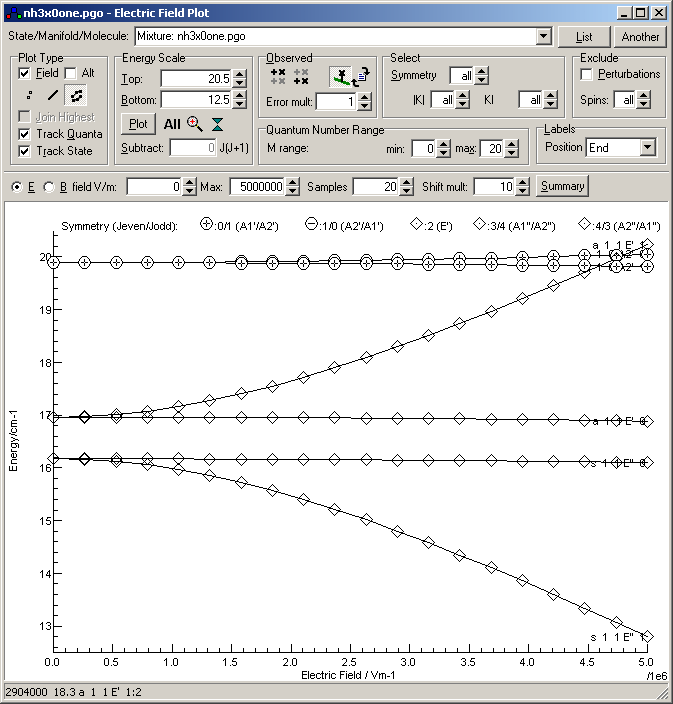Molecule Types Symmetric Tops Samples

# The pure rotational spectrum of the ground state of NH3/ND3 and the Stark effect

## NH3

The data files given here were constructed by fitting to the line positions and intensities in the HITRAN 2008 (http://www.hitran.com) database. The fit was restricted to J < 16, and is sufficient to reproduce the line positions to better than 0.001 cm-1 and the intensities to better than 10-20  cmcm-1 Molecule-1. There are two points that are slightly non-standard:

1. The point group used is D3h, rather than C3v. This is necessary to account for the inversion doubling in the ground state, which appears in the data file as two vibrational states:
• s/0+ with A1' symmetry
• a/0- with A2" symmetry
2. To fit the K = 3n levels requires three perturbation operators:
• <0+|J+-^6|0+> = J+6 + J-6
• <0+|J^2J+-^6|0+> = J2(J+6 + J-6)
• <0+|J+-^12|0+> = J+12 + J-12
Two separate data files are provided, with the required constants. The first is nh3x0.pgo which has the two inversion doublets in separate manifolds (s and a), with a transition moment acting between the two manifolds. This is a good starting point for simulating electronic spectra, where the transitions will typically be from one inversion doublet or other.

## The Stark Effect

The second file, nh3x0one.pgo, has the same constants in, but with the two inversion doublets in the same manifold. The resulting energy levels will be the same, but as the dipole moment now acts within the manifold, the Stark effect in the ground state can be simulated with this file. To give quick calculation times Jmax has been set to 5 for this file. The energy level plot window can then be used to produce a plot of energy against field, as for example:This shows the K = 1, M = 1 levels show a strong Stark effect, but the other levels show a rather weaker effect. The "Summary" button gives the following information:
`   M  Sym   #    g Population Name J K (kl) Sym M         Energy Linear         Dipole     Err  Quadratic       Err  Two Level Delta     C              Dipole2    Err   0    -   1    4 .660533316 a  0  0 A2"  0             0.7933 -5.20523347e-9 -.0309983 15.8% -1.0409798e-15 .02 %   0    -   2    2 .036129526 s  1  1 E"  0             16.1730 -1.47243024e-9 -.0087686 15.8% -2.9448374e-16 .002%   0    -   3    2 .032246486 a  1  1 E'  0             16.9632 -1.53137719e-9 -.0091197 15.8% -3.0627282e-16 .002%   0    -   4    4 .042329414 s  1  0 A2'  0            19.8899 3.241105275e-9 .01930147 15.8% 6.48153456e-16 .033%   1    -   1    2 .036129526 s  1  1 E"  1             16.1730 -7.02797997e-8 -.4185312 13.9% -1.3786935e-14 5.18% 16.583449 .82098935 -1.25126674e-7 -.7451561 .013%   1    -   2    2 .032246486 a  1  1 E'  1             16.9632 6.802667704e-8 .40511341 13.9% 1.33363101e-14 5.36% 16.583784 .75886528 1.190970869e-7 .70924861 .015%   1    -   3    4 .042329414 s  1  0 A2'  1            19.8899 -1.47290548e-9 -.0087715 15.8% -2.9457878e-16 .002%`
This indicates that the K = 1 levels are intermediate between a first and second order Stark effect, and the others are essentially showing a second order (quadratic) Stark effect. See External Fields - The Zeeman and Stark Effects for more information.

## ND3

A similar set of data files for ND3 is given below. The data sources are L Fusina and S N Murzin, J. Molec. Spectrosc. 167 464 (1994), L H Coudert and E Roueff, Astron. Astrophys. 449 855 (2006) and the ND3 linelist from the Cologne Database for Molecular Spectroscopy (CDMS).
• nd3x0.pgo, similar to the nh3x0.pgo file above. This was derived by from an unweighted fit to all lines in the CDMS linelist with J < 16, which gave an average error of 7×10-5 cm-1 and a maximum error of 0.003 cm-1. This has the two ground state inversion doublets in different manifolds, giving a simpler description than in the next file.
• nd3x0one.pgo, equivalent to the nh3x0one.pgo file above, with both ground state inversion doublets in the same manifold to allow the Stark effect to be simulated. This uses exactly the Hamiltonian and constants used in the Fusina and Murzin paper. This includes perturbations acting between the two inversion doublets, though note that the sign of the αJ and αK constants must be changed in PGOPHER to give consistent matrix elements. The line positions agree with the CDMS linelist to < 0.006 cm-1 (< 0.0005 cm-1 for J < 15). The operators mixing states are:
• <0-|JzJ+-^3|0+> = [J+3 + J-3, Jz]+ (term in α)
• <0+|J^2JzJ+-^3|0+> = J2[J+3 + J-3, Jz]+ (-term in αJ)
• <0+|Jz^3J+-^3|0+> = [J+3 + J-3, Jz3]+ (-term in αK)
• nd3x0hyp.pgo which has the hyperfine structure due to the nitrogen nucleus added to the simulation, giving a linelist consistent with the Coudert and Roueff paper.